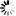Working...
This value you provided is not a number. Please try again.
This value you provided is not an integer. Please try again.
The value entered is not a valid Vanderbilt Medical Record Number (i.e. 4- to 9-digit number, excluding leading zeros). Please try again.
The value you provided must be within the suggested range.
The value you provided is outside the suggested range.
This value is admissible, but you may wish to verify.
The value entered must be a time value in the following format HH:MM within the range 00:00-23:59 (e.g., 04:32 or 23:19).
This field must be a 5 or 9 digit U.S. ZIP Code (like 94043). Please re-enter it now.
This field must be a 10 digit U.S. phone number (like 415 555 1212). Please re-enter it now.
This field must be a valid email address (like joe@user.com). Please re-enter it now.
The value you provided could not be validated because it does not follow the expected format. Please try again.
/^((29([-\/])02\3(\d{2}(||04|08)|(1600|200)))|((((0[1-9]|1\d|2[0-8])([-\/])(0[1-9]|1))|((29|30)([-\/])(0[13-9]|1))|(31([-\/])(0|1)))(\11|\15|\18)\d{4}))\$/
/^((02([-\/])29\3(\d{2}(||04|08)|(1600|200)))|((((0[1-9]|1)([-\/])(0[1-9]|1\d|2[0-8]))|((0[13-9]|1)([-\/])(29|30))|((0|1)([-\/])31))(\11|\15|\19)\d{4}))\$/
/^(((\d{2}(||04|08)|(1600|200))([-\/])02(\6)29)|(\d{4}([-\/])((0[1-9]|1)(\9)(0[1-9]|1\d|2[0-8])|((0[13-9]|1)(\9)(29|30))|((0|1)(\9)31))))\$/
/^((29([-\/])02\3(\d{2}(||04|08)|(1600|200)))|((((0[1-9]|1\d|2[0-8])([-\/])(0[1-9]|1))|((29|30)([-\/])(0[13-9]|1))|(31([-\/])(0|1)))(\11|\15|\18)\d{4})) (\d|[0-1]\d|[0-3]):[0-5]\d\$/
/^((02([-\/])29\3(\d{2}(||04|08)|(1600|200)))|((((0[1-9]|1)([-\/])(0[1-9]|1\d|2[0-8]))|((0[13-9]|1)([-\/])(29|30))|((0|1)([-\/])31))(\11|\15|\19)\d{4})) (\d|[0-1]\d|[0-3]):[0-5]\d\$/
/^(((\d{2}(||04|08)|(1600|200))([-\/])02(\6)29)|(\d{4}([-\/])((0[1-9]|1)(\9)(0[1-9]|1\d|2[0-8])|((0[13-9]|1)(\9)(29|30))|((0|1)(\9)31)))) (\d|[0-1]\d|[0-3]):[0-5]\d\$/
/^((29([-\/])02\3(\d{2}(||04|08)|(1600|200)))|((((0[1-9]|1\d|2[0-8])([-\/])(0[1-9]|1))|((29|30)([-\/])(0[13-9]|1))|(31([-\/])(0|1)))(\11|\15|\18)\d{4})) (\d|[0-1]\d|[0-3])(:[0-5]\d){2}\$/
/^((02([-\/])29\3(\d{2}(||04|08)|(1600|200)))|((((0[1-9]|1)([-\/])(0[1-9]|1\d|2[0-8]))|((0[13-9]|1)([-\/])(29|30))|((0|1)([-\/])31))(\11|\15|\19)\d{4})) (\d|[0-1]\d|[0-3])(:[0-5]\d){2}\$/
/^(((\d{2}(||04|08)|(1600|200))([-\/])02(\6)29)|(\d{4}([-\/])((0[1-9]|1)(\9)(0[1-9]|1\d|2[0-8])|((0[13-9]|1)(\9)(29|30))|((0|1)(\9)31)))) (\d|[0-1]\d|[0-3])(:[0-5]\d){2}\$/
/^([_a-z0-9-']+)([.+][_a-z0-9-']+)*@([a-z0-9-]+)(\.[a-z0-9-]+)*(\.[a-z]{2,63})\$/i
/^[-+]?\b\d+\b\$/
/^[a-z]+\$/i
/^\d{10}\$/
/^[-+]?[0-9]*\.?[0-9]+([eE][-+]?[0-9]+)?\$/
/^-?\d+,\d\$/
/^-?\d+\.\d\$/
/^-?\d+,\d{2}\$/
/^-?\d+\.\d{2}\$/
/^-?\d+,\d{3}\$/
/^-?\d+\.\d{3}\$/
/^-?\d+,\d{4}\$/
/^-?\d+\.\d{4}\$/
/^[-+]?[0-9]*,?[0-9]+([eE][-+]?[0-9]+)?\$/
/^(\(0[2-8]\)|0[2-8])\s*\d{4}\s*\d{4}\$/
/^(?:\(?([2-9]0[1-9]|[2-9]1[02-9]|[2-9][2-9][0-9])\)?)\s*(?:[.-]\s*)?([2-9]1[02-9]|[2-9][02-9]1|[2-9][02-9]{2})\s*(?:[.-]\s*)?([0-9]{4})(?:\s*(?:#|x\.?|ext\.?|extension)\s*(\d+))?\$/
/^\d{4}\$/
/^[ABCEGHJKLMNPRSTVXY]{1}\d{1}[A-Z]{1}\s*\d{1}[A-Z]{1}\d{1}\$/i
/^\d{3}-\d\d-\d{4}\$/
/^([0-9]|[0-1][0-9]|[0-3]):([0-5][0-9])\$/
/^[0-5]\d:[0-5]\d\$/
/^[0-9]{4,9}\$/
/^\d{5}(-\d{4})?\$/